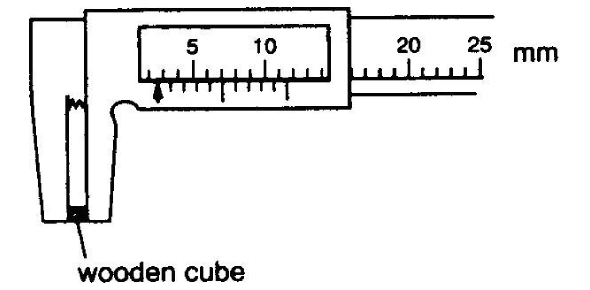10 Questions | Total Attempts: 15940SettingsTake our measurement reading quiz with informative questions and answers to find out exactly how much you remember or know about reading the measurements on many different devices. You will need to score 7/10 to pass this quiz. Measurement is something that we cannot live without and we have been doing it even before the formalization of measurement quantities, but it was very troublesome. Hence, with this formalization now complete, we can establish tests just like this one. All the best!

• 1.
The diagram shows part of a micrometer screw gauge. What is the reading shown?
• A.

5.31 mm

• B.

5.79 mm

• C.

5.81 mm

• D.

6.31 mm

• 2.
The diagram shows a micrometer. Which reading is shown?
• A.

2.23 mm

• B.

2.73 mm

• C.

3.23 mm

• D.

5.23 mm

• 3.
The diagram below shows part of a micrometer screw gauge. What is the reading?
• A.

5.37 mm

• B.

5.537 mm

• C.

5.87 mm

• D.

6.37 mm

• 4.
The diagram below shows part of a micrometer screw gauge. What is the reading shown?
• A.

4.01 mm

• B.

4.51 mm

• C.

5.00 mm

• D.

4.50 mm

• E.

4.60 mm

• 5.
The precision of a vernier calipers is _______ millimetres?
• 6.
The precision of a micrometer screw gauge is 0.001 cm
• A.

True

• B.

False

• 7.
A student used a vernier caliper to measure the length of a small wooden block. The diagram shows an enlargement of the caliper scales. What reading was recorded?
• A.

1.06 cm

• B.

1.16 cm

• C.

1.26 cm

• D.

1.36 cm

• 8.
The diagram shows part of a vernier calipers.
• A.

3.47 cm

• B.

3.67 cm

• C.

4.37 cm

• D.

4.57 cm

• 9.
Vernier calipers are used to measure a wooden cube as shown. What is the width of the cube as recorded by the vernier scale?
• A.

1.6 mm

• B.

2.6 mm

• C.

8.0 mm

• D.

11.4 mm

• 10.
A student used a vernier caliper to measure the diameter of a wooden cylinder. The diagram shows an enlargement of the caliper scales. What reading was recorded?
• A.

2.40 cm

• B.

1.64 cm

• C.

0.62 cm

• D.

0.42 cm

Related TopicsBack to top